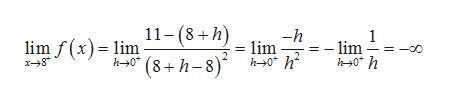# Estimate the one-sided limits for the function below. f(x)=(11−x)/(x−8)^2 lim f(x)=x-->8+ limf(x)=x-->8-

Question
1 views

Estimate the one-sided limits for the function below.

f(x)=(11−x)/(x−8)^2

lim f(x)=

x-->8+

limf(x)=

x-->8-

check_circle

Step 1

Given function is

Step 2

To find right hand limit , we substitute x=8+h, where h is positivehelp_outlineImage Transcriptionclose11-(8+h) -h - lim 1 lim h0 h lim f(x) lim h0 |(8+h-8) 0 h fullscreen
Step 3

To find left hand limit , we substitu...

### Want to see the full answer?

See Solution

#### Want to see this answer and more?

Solutions are written by subject experts who are available 24/7. Questions are typically answered within 1 hour.*

See Solution
*Response times may vary by subject and question.
Tagged in

### Other